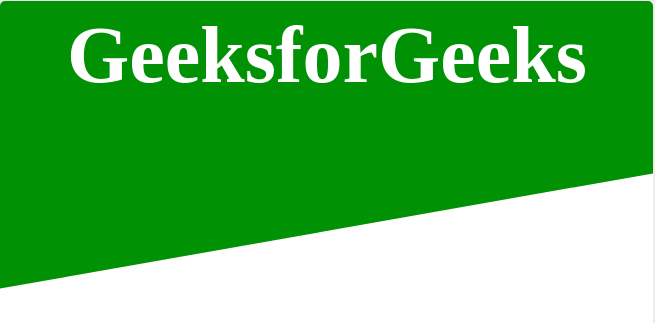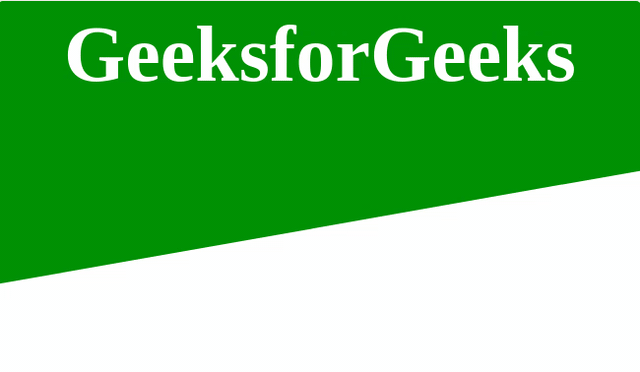Open In App

# How to create Skewed Background with hover effect using HTML and CSS?The skewed background or you can say an angel color shade background can be created by using HTML and CSS. This background can be used as a cover pic of your website that will be attractive. In this article, we will create a simple skewed background. We will divide the article into two sections in the first section we will create the structure and in the second section, we decorate the structure.

Creating structure: In this section, we will create the structure by using only simple HTML codes.

• HTML Code: By using the HTML <section> tag we will create the section for our skewed background which will have a HTML <div> tag inside of it.

 ```<``html``>`` ` `<``head``>``    ``<``meta``>``    ``<``title``>``        ``Skewed Background using HTML and CSS``    `````` ` `<``body``>``    ``<``section``>``        ``<``div` `class``=``"content"``>``            ``<``h2``>GeeksforGeeks``        ````    ```` ` ``` ` `                    `

Designing structure: In this section we will decorate the pre-created structure with the help of CSS.

• CSS Code: In this section first we will use some CSS properties to design the background and then we will use the skew property of the CSS which skews an element along the x and Y axis by the given angles.

 ``

Final Code: It is the combination of the above two code sections by combining the above two sections we can achieve the skewed background.

• Program:

 ```<``html``>`` ` `<``head``>``    ``<``meta``>``    ``<``title``>``        ``Skewed Background using HTML and CSS``    ``````<``style``>``    ``body {``        ``margin: 0;``        ``padding: 0;``        ``font-family: serif;``    ``}``     ` `    ``section:hover {``          ``background-image: linear-gradient(to left, green , yellow);``          ``transition-time: 5s;``    ``}``    ``section {``        ``display: flex;``        ``background: green;``        ``height: 350px;``        ``justify-content: center;``        ``align-items: center;``        ``transform: skew(0deg, -10deg) translateY(-120px);``    ``}``     ` `    ``.content {``        ``margin: 0;``        ``padding: 0;``        ``position: relative;``        ``max-width: 900px;``        ``transform: skew(0deg, 10deg);``        ``text-align: center;``    ``}``     ` `    ``.content h2 {``        ``color: #fff;``        ``font-size: 80px;``    ``}```` ` `<``body``>``    ``<``section``>``        ``<``div` `class``=``"content"``>``            ``<``h2``>GeeksforGeeks``        ````    ```` ` ``` ` ```  `

• Output: